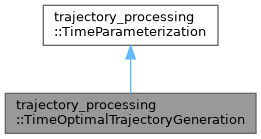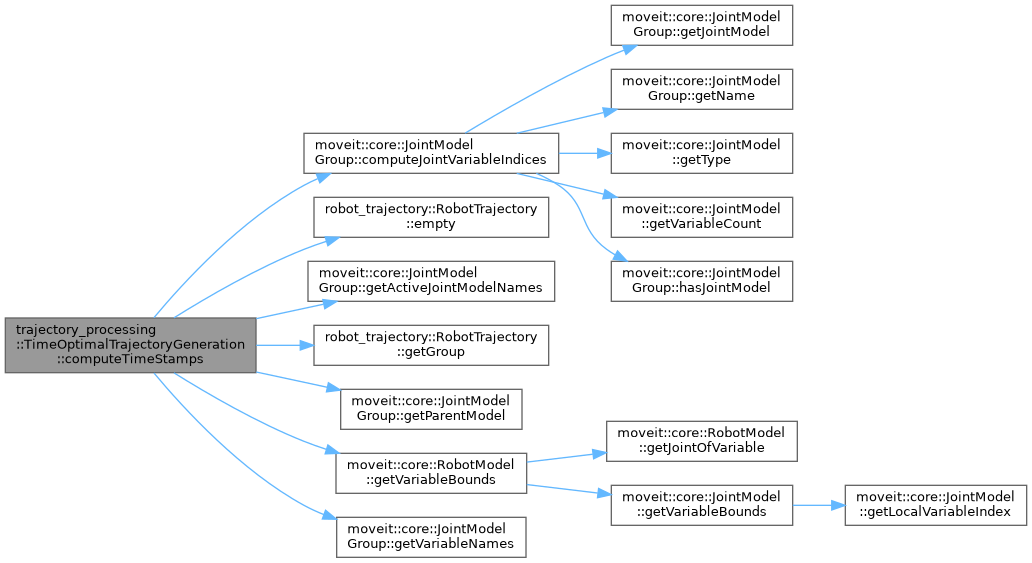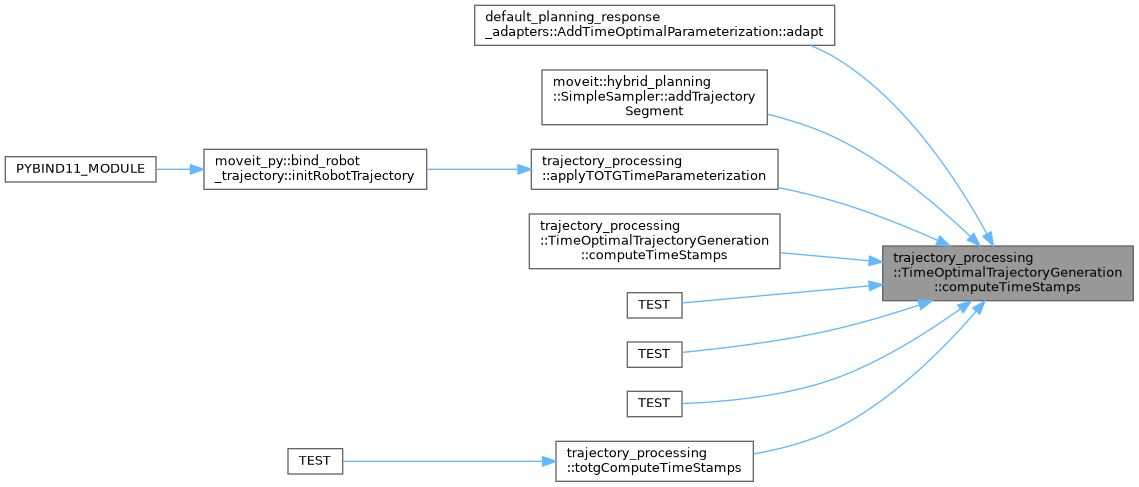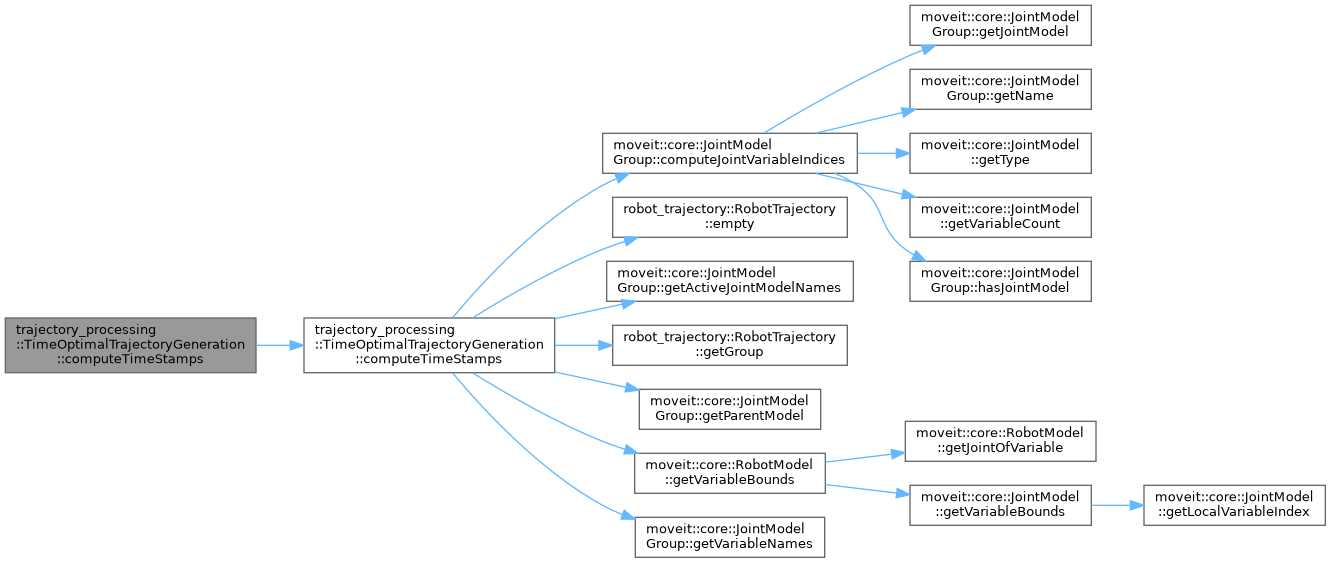moveit2 The MoveIt Motion Planning Framework for ROS 2.
trajectory_processing::TimeOptimalTrajectoryGeneration Class Reference

`#include <time_optimal_trajectory_generation.h>`

Inheritance diagram for trajectory_processing::TimeOptimalTrajectoryGeneration:[legend]
Collaboration diagram for trajectory_processing::TimeOptimalTrajectoryGeneration:[legend]

## Public Member Functions

TimeOptimalTrajectoryGeneration (const double path_tolerance=0.1, const double resample_dt=0.1, const double min_angle_change=0.001)

bool computeTimeStamps (robot_trajectory::RobotTrajectory &trajectory, const double max_velocity_scaling_factor=1.0, const double max_acceleration_scaling_factor=1.0) const override
Compute a trajectory with waypoints spaced equally in time (according to resample_dt_). Resampling the trajectory doesn't change the start and goal point, and all re-sampled waypoints will be on the path of the original trajectory (within path_tolerance_). However, controller execution is separate from MoveIt and may deviate from the intended path between waypoints. path_tolerance_ is defined in configuration space, so the unit is rad for revolute joints, meters for prismatic joints. More...

bool computeTimeStamps (robot_trajectory::RobotTrajectory &trajectory, const std::unordered_map< std::string, double > &velocity_limits, const std::unordered_map< std::string, double > &acceleration_limits, const double max_velocity_scaling_factor=1.0, const double max_acceleration_scaling_factor=1.0) const override
Compute a trajectory with waypoints spaced equally in time (according to resample_dt_). Resampling the trajectory doesn't change the start and goal point, and all re-sampled waypoints will be on the path of the original trajectory (within path_tolerance_). However, controller execution is separate from MoveIt and may deviate from the intended path between waypoints. path_tolerance_ is defined in configuration space, so the unit is rad for revolute joints, meters for prismatic joints. More...

bool computeTimeStamps (robot_trajectory::RobotTrajectory &trajectory, const std::vector< moveit_msgs::msg::JointLimits > &joint_limits, const double max_velocity_scaling_factor=1.0, const double max_acceleration_scaling_factor=1.0) const override
Compute a trajectory with waypoints spaced equally in time (according to resample_dt_). Resampling the trajectory doesn't change the start and goal point, and all re-sampled waypoints will be on the path of the original trajectory (within path_tolerance_). However, controller execution is separate from MoveIt and may deviate from the intended path between waypoints. path_tolerance_ is defined in configuration space, so the unit is rad for revolute joints, meters for prismatic joints. More...Public Member Functions inherited from trajectory_processing::TimeParameterization
TimeParameterization ()=default

TimeParameterization (const TimeParameterization &)=default

TimeParameterization (TimeParameterization &&)=default

TimeParameterizationoperator= (const TimeParameterization &)=default

TimeParameterizationoperator= (TimeParameterization &&)=default

virtual ~TimeParameterization ()=default

## Detailed Description

Definition at line 180 of file time_optimal_trajectory_generation.h.

## ◆ TimeOptimalTrajectoryGeneration()

 trajectory_processing::TimeOptimalTrajectoryGeneration::TimeOptimalTrajectoryGeneration ( const double path_tolerance = `0.1`, const double resample_dt = `0.1`, const double min_angle_change = `0.001` )

Definition at line 876 of file time_optimal_trajectory_generation.cpp.

## ◆ computeTimeStamps() [1/3]

 bool trajectory_processing::TimeOptimalTrajectoryGeneration::computeTimeStamps ( robot_trajectory::RobotTrajectory & trajectory, const double max_velocity_scaling_factor = `1.0`, const double max_acceleration_scaling_factor = `1.0` ) const
overridevirtual

Compute a trajectory with waypoints spaced equally in time (according to resample_dt_). Resampling the trajectory doesn't change the start and goal point, and all re-sampled waypoints will be on the path of the original trajectory (within path_tolerance_). However, controller execution is separate from MoveIt and may deviate from the intended path between waypoints. path_tolerance_ is defined in configuration space, so the unit is rad for revolute joints, meters for prismatic joints.

Parameters
 [in,out] trajectory A path which needs time-parameterization. It's OK if this path has already been time-parameterized; this function will re-time-parameterize it. max_velocity_scaling_factor A factor in the range [0,1] which can slow down the trajectory. max_acceleration_scaling_factor A factor in the range [0,1] which can slow down the trajectory.

Implements trajectory_processing::TimeParameterization.

Definition at line 882 of file time_optimal_trajectory_generation.cpp.

Here is the call graph for this function:Here is the caller graph for this function:## ◆ computeTimeStamps() [2/3]

 bool trajectory_processing::TimeOptimalTrajectoryGeneration::computeTimeStamps ( robot_trajectory::RobotTrajectory & trajectory, const std::unordered_map< std::string, double > & velocity_limits, const std::unordered_map< std::string, double > & acceleration_limits, const double max_velocity_scaling_factor = `1.0`, const double max_acceleration_scaling_factor = `1.0` ) const
overridevirtual

Compute a trajectory with waypoints spaced equally in time (according to resample_dt_). Resampling the trajectory doesn't change the start and goal point, and all re-sampled waypoints will be on the path of the original trajectory (within path_tolerance_). However, controller execution is separate from MoveIt and may deviate from the intended path between waypoints. path_tolerance_ is defined in configuration space, so the unit is rad for revolute joints, meters for prismatic joints.

Parameters
 [in,out] trajectory A path which needs time-parameterization. It's OK if this path has already been time-parameterized; this function will re-time-parameterize it. velocity_limits Joint names and velocity limits in rad/s acceleration_limits Joint names and acceleration limits in rad/s^2 max_velocity_scaling_factor A factor in the range [0,1] which can slow down the trajectory. max_acceleration_scaling_factor A factor in the range [0,1] which can slow down the trajectory.

Implements trajectory_processing::TimeParameterization.

Definition at line 984 of file time_optimal_trajectory_generation.cpp.

Here is the call graph for this function:## ◆ computeTimeStamps() [3/3]

 bool trajectory_processing::TimeOptimalTrajectoryGeneration::computeTimeStamps ( robot_trajectory::RobotTrajectory & trajectory, const std::vector< moveit_msgs::msg::JointLimits > & joint_limits, const double max_velocity_scaling_factor = `1.0`, const double max_acceleration_scaling_factor = `1.0` ) const
overridevirtual

Compute a trajectory with waypoints spaced equally in time (according to resample_dt_). Resampling the trajectory doesn't change the start and goal point, and all re-sampled waypoints will be on the path of the original trajectory (within path_tolerance_). However, controller execution is separate from MoveIt and may deviate from the intended path between waypoints. path_tolerance_ is defined in configuration space, so the unit is rad for revolute joints, meters for prismatic joints.

Parameters
 [in,out] trajectory A path which needs time-parameterization. It's OK if this path has already been time-parameterized; this function will re-time-parameterize it. joint_limits Joint names and corresponding velocity limits in rad/s and acceleration limits in rad/s^2 max_velocity_scaling_factor A factor in the range [0,1] which can slow down the trajectory. max_acceleration_scaling_factor A factor in the range [0,1] which can slow down the trajectory.

Implements trajectory_processing::TimeParameterization.

Definition at line 961 of file time_optimal_trajectory_generation.cpp.

Here is the call graph for this function:The documentation for this class was generated from the following files: